##### Permutations and Combinations

Permutations and subsets are the most basic combinatorial objects. DiscreteMath`Combinatorica` provides functions for constructing objects both randomly and deterministically, to rank and unrank them, and to compute invariants on them. Here we provide examples of some of these functions in action.

These permutations are generated in minimum change order, where successive permutations differ by exactly one transposition. The builtşin generator Permutations constructs permutations in lexicographic order.

`MinimumChangePermutations[{a,b,c,d}]`
`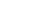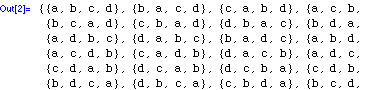`

The ranking function illustrates that the builtşin function Permutations uses lexicographic sequencing.

`Map[RankPermutation, Permutations[{1,2,3,4}]]`
`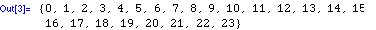`

With $3! = 6$ distinct permutations of three elements, within 20 random permutations we are likely to see all of them. Observe that it is unlikely for the first six permutations to all be distinct.

`Table[RandomPermutation, {20}]`
``

A fixed point of a permutation $p$ is an element in the same position in $p$ as in the inverse of $p$. Thus, the only fixed point in this permutation is 7.

`InversePermutation[{4,8,5,2,1,3,7,6}]`
`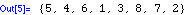`

The identity permutation consists of $n$ singleton cycles or fixed points.

`ToCycles[{1,2,3,4,5,6,7,8,9,10}]`
``

The classic problem in Polya theory is counting how many different ways necklaces can be made out of $k$ beads, when there are $m$ different types or colors of beads to choose from. When two necklaces are considered the same if they can be obtained only by rotating the beads (as opposed to turning the necklace over), the symmetries are defined by $k$ permutations, each of which is a cyclic shift of the identity permutation. When a variable is specified for the number of colors, a polynomial results.

`Polya[Table[ RotateRight[Range,i], {i,8}], m]`
`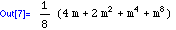`

The number of inversions in a permutation is equal to that of its inverse.

`(p=RandomPermutation; {Inversions[p], Inversions[InversePermutation[p]]})`
`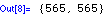`

Generating subsets incrementally is efficient when the goal is to find the first subset with a given property, since every subset need not be constructed.

`Table[NthSubset[n,{a,b,c,d}], {n,0,15}]`
`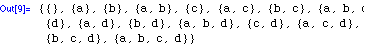`

In a Gray code, each subset differs in exactly one element from its neighbors. Observe that the last eight subsets all contain $4$, while none of the first eight do.

`GrayCode[{1,2,3,4}]`
`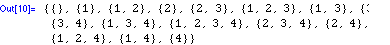`

A $k$şsubset is a subset with exactly $k$ elements in it. Since the lead element is placed in first, the $k$şsubsets are given in lexicographic order.

`KSubsets[{1,2,3,4,5},3]`
`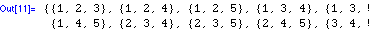`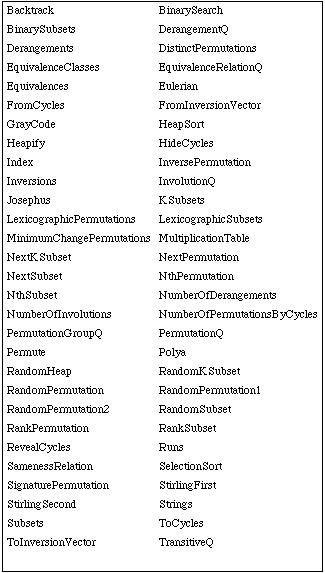Combinatorica functions for permutations and combinations.